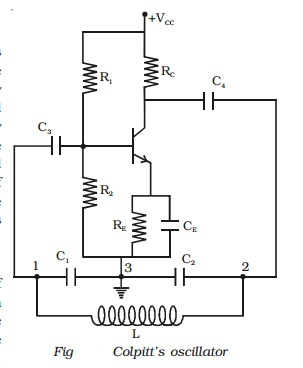Home | | Physics | Colpitt's oscillator

# Colpitt's oscillatorA transistor can work as an LC oscillator to produce undamped oscillations of any desired frequency, if tank and feedback circuits are properly connected to it. There are different LC oscillators used in electronic circuits, of which, the working principle of Colpitt's oscillator is discussed here.

LC oscillators

A transistor can work as an LC oscillator to produce undamped oscillations of any desired frequency, if tank and feedback circuits are properly connected to it. There are different LC oscillators used in electronic circuits, of which, the working principle of Colpitt's oscillator is discussed here.

Colpitt's oscillator

The circuit diagram of Colpitt's oscillator is shown in Fig. The resistance R1, R2 and RE provide the sufficient bias for the circuit The frequency determining network is the parallel resonant circuits consisting of capacitors C1, C2 and the inductor L. The junction of C1 and C2 is earthed. The function of the capacitor C4 is to block d.c and provide an a.c. path from the collector to the tank circuit. The voltage developed across C1 provides the positive feedback for sustained oscillations.

Working

When the collector supply voltage is switched on, a transient current is produced in the tank circuit and damped harmonic oscillations are produced. The oscillations across C1 are applied to the base emitter junction and appear in the amplified form in the collector circuit. If terminal 1 is at positive potential with respect to terminal 3 at any instant, then terminal 2 will be at negative potential with respect to 3, since 3 is grounded. Hence points 1 and 2 are 180o out of phase. The amplifier produces further phase shift of 180o. Thus the total phase shift is 360o. In other words, energy supplied to the tank circuit is in phase with the oscillations and if A = 1, oscillations are sustained in the circuit.

The frequency of oscillations is given by f = 1/2πLC

Where C= C1C2/C1+C2

F=1/2π rt((C1+C2)LC1C2)Study Material, Lecturing Notes, Assignment, Reference, Wiki description explanation, brief detail
11th 12th std standard Class Physics sciense Higher secondary school College Notes : Colpitt's oscillator |